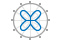# ezpolar

•## 语法

```ezpolar(fun) ezpolar(fun,[a,b]) ezpolar(axes_handle,...) h = ezpolar(...) ```

## 说明

`ezpolar(fun)` 在默认域 0 < `theta` < 2π 中绘制极坐标曲线 `rho = fun(theta)`

`fun` 可以是函数句柄、字符向量或字符串（请参阅提示部分）。

`ezpolar(fun,[a,b])` 绘制 `a` < `theta` < `b``fun`

`ezpolar(axes_handle,...)` 将图形绘制到带有句柄 `axes_handle` 的坐标区中，而不是当前坐标区 (`gca`) 中。

`h = ezpolar(...)` 将句柄返回给 `h` 中的线对象。

## 示例

```figure ezpolar('1+cos(t)')```## 提示

### 以字符向量或字符串形式传递函数

`t.^2.*cos(t)`

`ezpolar('t^2*cos(t)')`

### 传递函数句柄

```fh = @(t) t.^2.*cos(t); ezpolar(fh)```

### 传递附加参数

```function s = myfun(t,k1,k2) s = sin(k1*t).*cos(k2*t);```

`ezpolar(@(t)myfun(t,2,3))`